## Expectation Maximization with Gaussian Mixture Models

Learn how to model multivariate data with a Gaussian Mixture Model. For training this model, we use a technique called Expectation Maximization.

## One dimensional mixture

Series: Expectation Maximization with Gaussian Mixture Models:

1. Introduction
2. One dimensional mixture

In the previous part of this series, I introduced you to the idea of learning a single Gaussian given some data. It seemed to work just fine and was able to learn the distribution of data pretty well. As we increase the number of datapoints available (maybe by letting the physical sensors run for a longer duration), we would get a progressively a better learned model.

However, most data isn't as simple as a single Gaussian. Take a look at this data distribution, you can clearly see "clumps" of data. There is no way a single Gaussian (something with a single peak) can model this accurately. A mixture of Gaussians is necessary for representing such data. And we'll do exactly that.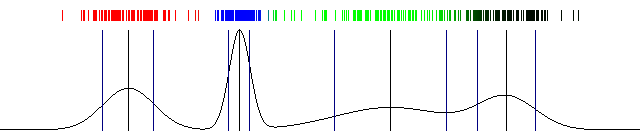An example of a more complex data distribution

## Expectation Maximization

With multiple Gaussian curves to learn, we now have to turn to the EM algorithm. Let's take a look at the math behind it to get started. Let's start with a single Gaussian function: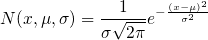To have a mixture or a linear combination of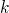Gaussians, the equation would look something like this: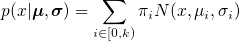Before you get bogged down in all those symbols, let me explain what they mean. The probability now comtains a summation - that's the linear combination. We have a weighting factor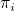per Gaussian curve. Also note that we now use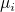and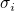when calculating the probability. We pass in the corresponding mean and standard deviation.

On the left hand side, we now have bolded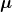and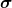. These represent a vector of means and standard deviations.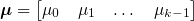This is just for convenience. Imagine passing 50 variables to a function - that would be inconvenient. However, passing an array is convenient. This is the mathematical equivalent.

### Hidden variables

The mean and standard deviations can be inferred from the datapoints directly. We did that in the previous part - you simply plug in the formulae and calculate the mean and standard deviation. However, in our new model, we have a so called Hidden variable which is(the weighting factor for each Gaussian).

This is a new variable we have introduced and isn't observable from the data. How do we learn it if we can't even observe it? That's where Expectation Maximization comes into picture.

The goal of this technique is to assume some initial mean, standard deviation and pi values and iteratively improve the estimate. Every single iteration is made up of two steps - the so E step and the M step.

The E step: This is the expectation part. Using the current mean and standard deviation guess, we calculate probabilities. We calculate these values for each Gaussian. This helps us predict which Gaussian is responsble for which datapoint (called the responsibility).

The M step: This is the maximization part. Using the responsibilities in the previous step, we update the mean and standard deviations. These calculations are somewhat analogous to their single Gaussian equivalents.

Keep doing these two steps one after the other. Eventually, the values of the mean and standard deviation will stabilize - that will be the learned model.

Values stabilizing at a specific value is called convergence and is detectable. We'll look at when to stop the iterations once we actually finish the implementation of a multi-dimensional GMM.

### The Expectation Step

The expectation step is for calculating responsibilities.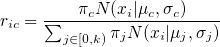Let's look at the numerator and denominator of this equation separately. The responsibility is calculated for each data point and for every cluster. So if you have 100 datapoints and have a mixture of five Gaussians, you would have to calculate 500 numbers. In practice, it is useful to think of this as a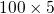array or matrix.

So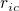is the responsibility of datapointwith respect to the Gaussian curve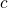(out ofpossible curves). This is represented by the numerator - which is just the probability of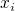under theGaussian curve. We multiply it by the weight as well - since this is a linear combination and we need to account for the constant factor.

Now let's look at the denominator. It is simply the sum of probabilties of the data pointunder all the Gaussian curves. This means, it acts as a normalizing factor - ensuring that the value ofis between zero and one for all curves. This ensures that the value we get is a valid probability.

And that's it - the E step just involves computing this quantity called responsibility!

### The Maximization Step

Now that we have the responsibilities for every datapoint with respect to each Gaussian curve, we can use this to improve our guess of each curve's mean, standard deviation and the weighting factor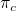.

To calculate the new mean for every curve, we have: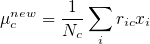This is very similar to learning the mean of a single Gaussian curve. The idea is, you sum up all the points and divide them by the number of points. The only difference here is that we're using the "responsibility" to account for membership in a Gaussian curve.

In a single Gaussian curve, every datapoint is a member of the curve - thus the responsibility is 1. However for multiple Gaussians, a single datapoint might contribute more to curve A rather than curve B. Ths responsibility accounts for this.

The denominator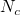takes into account this as well.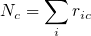For a single Gaussian curve, this would simply be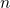- the number of points in the dataset. However, for multiple curves, each datapoint has a different responsibility - and thus adds only a fraction to the total point count for curve.

We have a very similar equation for calculating the standard deviation as well: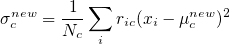Again, the idea is that each point only partially contributes to each curve. Similarly, we can calculate the new values ofas well: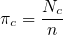Here,is the total number of datapoints in the dataset (irrespective of which curve they belong to).

### Initial guess to start EM

We need to kickstart this algorithm with some mean and standard deviation values. For this article, we'll stick to manually assigning these values. It is possible to use a better / smarter approach (for example using k-means or using PCA to figure out the main regions in a dataset).

## Learning Gaussian Mixtures with OpenCV

With all this knowledge, we're now ready to actually implement it now.

OpenCV 3+ comes with Gaussian Mixture Models built right into the library. Look for the `GMM` class.

### Step 1: Making an initial guess

Let's start out by making a new OpenCV project. I'll be using the `generate_1d_data` function from the previous part. Let's create a new function that generates an initial guess for us.

```float* get_data_means_var(float* data, int count_per_component, int num_components) {
float* ret = new float[2*num_components];

for(int i=0;i<2*num_components;i+=2) {
ret[i] = 640*i/(2.0f*num_components) + 120;
ret[i+1] = 1000;
}

return ret;
}
```

The idea here is simply, we're returning a bunch of means and a hard-coded variance of 1000. It is possible to find "better" means and variances (like using the technique here) - but for thus tutorial, we'll stick to only learning about the EM algorithm.

Now we'll define a new function that will run the entire EM algorithm for us.

```void run_gaussian_mixture_1d() {
const int num_components = 2;
const int count_per_component = 100;
float *data = new float[num_components*count_per_component];

float mean = {120, 480, 240, 360, 320};
float std = {20, 30, 10, 40, 5};
```

For this part, I'm hard-coding the original mean and std to some neatly spaced values. We're defining the number of components and allocating sufficient memory to store 100 data points for each component.

```    for(int i=0;i<num_components;i++) {
float* new_data = generate_1d_data(mean[i], std[i], count_per_component);
memcpy(data+(count_per_component*i), new_data, sizeof(float)*count_per_component);
}
```

Here, we use the same function from the previous part to generate points from the i'th component's mean and standard deviation.

```    float* initial_guess = get_data_means_var(data, count_per_component, num_components);
```

I create a new function that returns an initial guess for us. For this tutorial, I've arranged this array like this: `[mean0, var0, mean1, var1, ..]`.

```    int total = num_components * count_per_component;
float* responsibility = new float[num_components*total];
float* pi             = new float[num_components];
float* learned_means  = new float[num_components];
float* learned_std    = new float[num_components];
```

Before we can start doing the iterations, we need to allocate enough memory for all the steps. This is where I do this.

```    for(int i=0;i<num_components;i++) {
learned_means[i] = initia_guess[2*i];
learned_std[i] = sqrt(initial_guess[2*i+1]);
pi[i] = 1.0f/num_components;
}
```

Here, I copy our initial guess into the `learned_means` and `learned_std` arrays. I also initial the weighting factors of the Gaussians - I start out with the assumption that each Gaussian is weight equally at the beginning.

### Step 2: The E step

We all this setup, we're now ready for doing the E step - which is about calculating responsibilities for each datapoint with respect to each Gaussian curve.

```    for(int iter=0;iter<100;iter++) {
// E step
for(int i=0;i<total;i++) {
float total_prob = 0.0f;
for(int j=0;j<num_components;j++) {
float prob = pi[j] * calculate_1d_probability(data[i], learned_means[j], learned_Std[j]);
total_prob += prob;
responsibility[i*num_components+j] = prob;
}

if(total_prob > 0) {
for(int j=0;j<num_components;j++) {
responsibility(i*num_components + j] /= total_prob;
}
}
}
```

There are a few things happening here. We're running the EM steps 100 times (the outermost `for` loop). The second for loop (with variable `i`) loops through all datapoints. The third for loop with variable `j` loops over the individual Gaussian curves (the components). Using these two for loops, we can calculate the entire responsibility matrix.

Another thing we do is normalize the responsibilities for each datapoint. This is done only if the total probability is greater than 0 - otherwise you end up with a NaN values in the responsibility matrix - which isn't useful.

We also used a function that we've not defined yet - `calculate_1d_probability`. This function will simply be an implementation of the Gaussian equation. You'll see its implementation later!

### Step 3: The M step

Let's move onto the M part now. Here, we need to update the various parameters: `pi`, `learned_means` and `learned_std`. So let's start out with:

```        // M step
float* nc = new float[num_components];
memset(nc, 0, sizeof(float) * num_components);
for(int i=0;i<total;i++) {
for(int j=0;j<num_components;j++) {
nc[j] += responsibility[i*num_components+j];
}
}
```

This is simply the summation of all responsibilities per Gaussian. So `nc` would give me the sum of all responsibilities for Gaussian 0. The next step is calculating the updated value of `pi`.

```        for(int j=0;j<num_components;j++) {
pi[j] = nc[j]/total;
}
```

This is simply an implementation of the equation mentioned earlier. In cases where there is only a single Gaussian, `nc[j]` would be equal to `total` and thus `pi` would be 1.0. In other cases, it would be a number between 0 and 1.

```        for(int j=0;j<num_components;j++) {
learned_means[j] = 0;
for(int i=0;i<total;i++) {
learned_means[j] += responsbility[i*num_components+j] * data[i];
}

if(nc[j] > 0) {
learned_means[j] /= nc[j];
}

for(int i=0;i<total;i++) {
float diff = data[i] - learned_means[j];
learned_std[j] += responsibility[i*num_components+j] * diff * diff;
}

if(nc[j] > 0) {
learned_std[j] /= nc[j];
}

learned_std[j] = sqrt(learned_std[j]);
}
```

Again, just an implementation of a bunch of equations. THe idea is to use the responsibilities and calculate the new mean and standard deviation. Pretty straightforward, I think!

### Step 4: Drawing the graphs

Finally, we can now draw what we've just learned.

```        const int graph_width = 640;
const int graph_height = 140;
cv::Mat graph(graph_height, graph_width, CV_8UC3, cv::Scalar(255, 255, 255));
draw_1d_data_mixture(graph, data, responsibility, count_per_component, num_componnets);
draw_1d_gaussian_mixture(graph, learned_means, learned_std, pi, num_components);

for(int j=0;j<num_components;j++) {
printf("Iteration %04d\n", iter);
print("Learned #%d - mean = %f, td = %f, pi =%f\n", j, learned_means[j], learned_std[j], pi[j]);
}

char file;
sprintf(file, "./mixture-gaussian-iter%02d.png", iter);
cv::imwrite(file, graph);
}
return
}
```

We've used a bunch of functions here that we haven't yet created. In the end, I save out the graph into a file so you can visualize how the various curves move around over time.

### Defining all functions

We have quite a backlog of functions we haven't yet defined. Let's define them. Let's start out with calculating the 1D probability given a standard deviation and a mean:

```float calculate_1d_probability(float value, float mean, float std) {
float ret = 0.0f;

float frac = 1.0f / (std * sqrt(2 * M_PI));
float power = -0.5f * pow((value - mean) / std, 2.0);

ret = frac * exp(power);

return ret;
}
```

This function simply implements the Gaussian function in one dimension: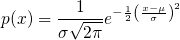For simplicity, I've split up the fraction on outside the exponentiation and the fraction inside the exp into two separate parts. Now onto some drawing functions.

```void draw_1d_draw_mixture(cv::Mat img, float* data, float* weights, int count_per_component, int num_components {
std::vector<cv::Scalar> palette;
palette.push_back(cv::Scalar(0, 0, 255));   // red
palette.push_back(cv::Scalar(255, 0, 0));
palette.push_back(cv::Scalar(0, 255, 0));
palette.push_back(cv::Scalar(0, 0, 0));
palette.push_back(cv::Scalar(192, 192, 192));
```

I wanted to see how the membership (responsibilities) of the various datapoints changed over time. To do this, I created a small palette of colors. Datapoints belonging to curve 0 would be assigned the first color (red - because the colors are BGR), the second would be blue and so on.

If there are partial membership, the colors would blend together - 0.4red + 0.6blue. It would be a nice visualization. Now let's get to the actual drawing.

```    int total = count_per_component * num_components;
for(int i=0;i<total;i++) {
float* wt = &weights[i*num_components];

cv::Scalar color = cv::Scalar(0);
for(int j=0;j<num_components;j++) {
color += wt[j] * palette[j];
}

float pt = data[i];
cv::line(img, cv::Point(pt, 10), cv::Point(pt, 20), color);
}
}
```

The array `wt` here is the responsibilities of the datapoint `data[i]`. We loop through the different components and combine the palette colors based on the responsibilities. And finally, we draw a line on the graph.

The next function we need to write is drawing the curves themselves. This is actually very simiar to the drawing code in the previous part. The only difference being that we have multiple curves now.

```void draw_1d_gaussian_mixture(cv::Mat img, float* mean, float* stddev, float* pi, int num_components) {
float* prob = new float[img.cols];
float* frac = new float[num_components];

for(int j=0;j<num_components;j++) {
frac[j] = 1.0 / (stddev[j] * sqrt(2*M_PI));
}
```

We start out by calculating the fraction that appears outside the Gaussian equation. Since it depends on the standard deviation, we calculate it per component.

```    float min_prob = FLT_MAX;
float max_prob = FLT_MIN;
memset(prob, 0, sizeof(float)*img.cols);

for(int x=0;x<img.cols;x++) {
for(int j=0;j<num_components;j++) {
prob[x] = pi[j] * frac[j] * exp(-0.5 * (x-mean[j])*(x-mean[j])/(stddev[j]*stddev[j]));
}

if(prob[x] > max_prob)
max_prob = prob[x];

if(prob[x] < min_prob)
min_prob = prob[x];
}
```

Here, we calculate the probability for data points between 0 and 640. Since our graph is conveniently 640px in width, calculating the probability at each pixel is good enough. We also store the maximum and minimum probabilty so we can draw the graph without clipping off the edges.

```    float prev = 0;
for(int x=0;x<img.cols;x++) {
float p = 30 + 100*(1-(prob[x]/(max_prob-min_prob)));
cv::line(img, cv::Point(x-1, prev), cv::Point(x, p), cv::Scalar(0));
prev = p;
}

for(int i=0;i<num_components;i++) {
cv::line(img, cv::Point(mean[i], 30), cv::Point(mean[i], 130), cv::Scalar(0));
cv::line(img, cv::Point(mean[i]-stddev[i], 30), cv::Point(mean[i]-stddev[i], 130), cv::Scalar(128));
cv::line(img, cv::Point(mean[i]+stddev[i], 30), cv::Point(mean[i]+stddev[i], 130), cv::Scalar(128));
}
}
```

Draw the actual probabilities and also draw the mean and standard deviation. And that's a wrap! Here's the result I got with the above code:100 iterations of Expectation Maximization and a one dimensional Gaussian Mixture Model (the image is animated)

## Wrap up

So now you've seen the EM algortihm in action and hopefully understand the big picture idea behind it. Until now, we've only been working with 1D Gaussians - primarily because of mathematical ease and they're easy to visualize.

In the next part, we'll look at extending this to multiple dimensions. This is where we need to start representing the Gaussian surface with a vector mean and a covariance matrix. They're even more powerful and more general purpose (for example, segmenting images using three dimensional RGB data).

## More in the series

This tutorial is part of a series called Expectation Maximization with Gaussian Mixture Models:

1. Introduction
2. One dimensional mixture

Utkarsh Sinha created AI Shack in 2010 and has since been working on computer vision and related fields. He is currently at Microsoft working on computer vision.